# Excel IF OR function with formula examples

The tutorial shows how to write an IF OR statement in Excel to check for various "this OR that" conditions.

IF is one of the most popular Excel functions and very useful on its own. Combined with the logical functions such as AND, OR, and NOT, the IF function has even more value because it allows testing multiple conditions in desired combinations. In this tutorial, we will focus on using IF-and-OR formula in Excel.

## IF OR statement in Excel

To evaluate two or more conditions and return one result if any of the conditions is TRUE, and another result if all the conditions are FALSE, embed the OR function in the logical test of IF:

IF(OR(condition1, condition2,...), value_if_true, value_if_false)

In plain English, the formula's logic can be formulated as follows: If a cell is "this" OR "that", take one action, if not then do something else.

Here's is an example of the IF OR formula in the simplest form:

`=IF(OR(B2="delivered", B2="paid"), "Closed", "Open")`

What the formula says is this: If cell B2 contains "delivered" or "paid", mark the order as "Closed", otherwise "Open".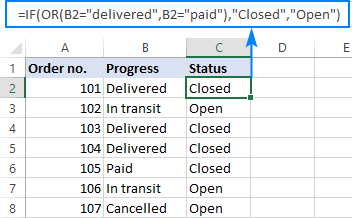In case you want to return nothing if the logical test evaluates to FALSE, include an empty string ("") in the last argument:

`=IF(OR(B2="delivered", B2="paid"), "Closed", "")`

The same formula can also be written in a more compact form using an array constant:

`=IF(OR(B2={"delivered","paid"}), "Closed", "")`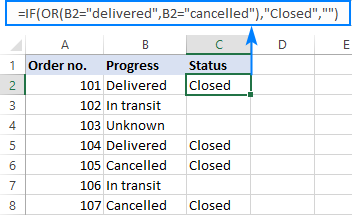In case the last argument is omitted, the formula will display FALSE when none of the conditions is met.

Note. Please pay attention that an IF OR formula in Excel does not differentiate between lowercase and uppercase characters because the OR function is case-insensitive. In our case, "delivered", "Delivered", and "DELIVERED", are all deemed the same word. If you'd like to distinguish text case, wrap each argument of the OR function into EXACT as shown in this example.

## Excel IF OR formula examples

Below you will find a few more examples of using Excel IF and OR functions together that will give you more ideas about what kind of logical tests you could run.

### Formula 1. IF with multiple OR conditions

There is no specific limit to the number of OR conditions embedded into an IF formula as long as it is in compliance with the general limitations of Excel:

• In Excel 2007 and higher, up to 255 arguments are allowed, with a total length not exceeding 8,192 characters.
• In Excel 2003 and lower, you can use up to 30 arguments, and a total length shall not exceed 1,024 characters.

As an example, let's check columns A, B and C for blank cells, and return "Incomplete" if at least one of the 3 cells is blank. The task can be accomplished with the following IF OR function:

`=IF(OR(A2="",B2="", C2=""),"Incomplete","")`

And the result will look similar to this: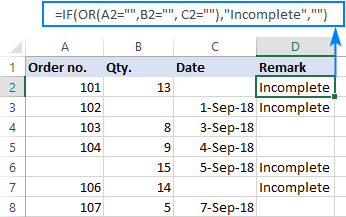### Formula 2. If a cell is this OR that, then calculate

Looking for a formula that can do something more complex than return a predefined text? Just nest another function or arithmetic equation in the value_if_true and/or value_if_false arguments of IF.

Say, you calculate the total amount for an order (Qty. multiplied by Unit price) and you want to apply the 10% discount if either of these conditions is met:

• in B2 is greater than or equal to 10, or
• Unit Price in C2 is greater than or equal to \$5.

So, you use the OR function to check both conditions, and if the result is TRUE, decrease the total amount by 10% (B2*C2*0.9), otherwise return the full price (B2*C2):

`=IF(OR(B2>=10, C2>=5), B2*C2*0.9, B2*C2)`

Additionally, you could use the below formula to explicitly indicate the discounted orders:

`=IF(OR(B2>=10, C2>=5),"Yes", "No")`

The screenshot below shows both formulas in action: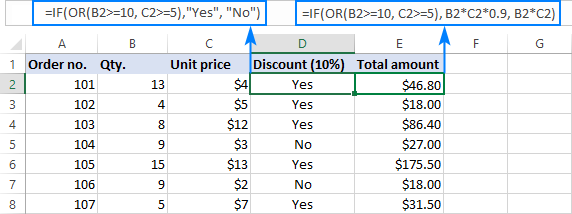### Formula 3. Case-sensitive IF OR formula

As already mentioned, the Excel OR function is case-insensitive by nature. However, your data might be case-sensitive and so you'd want to run case-sensitive OR tests. In this case, perform each individual logical test inside the EXACT function and nest those functions into the OR statement.

IF(OR(EXACT(cell,"condition1"), EXACT(cell,"condition2")), value_if_true, value_if_false)

In this example, let's find and mark the order IDs "AA-1" and "BB-1":

`=IF(OR(EXACT(A2, "AA-1"), EXACT(A2, "BB-1")), "x", "")`

As the result, only two orders IDs where the letters are all capital are marked with "x"; similar IDs such as "aa-1" or "Bb-1" are not flagged: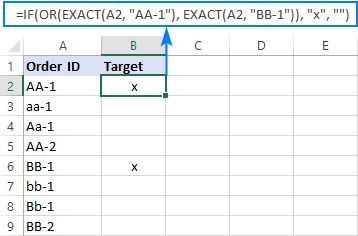### Formula 4. Nested IF OR statements in Excel

In situations when you want to test a few sets of OR criteria and return different values depending on the results of those tests, write an individual IF formula for each set of "this OR that" criteria, and nest those IF's into each other.

To demonstrate the concept, let's check the item names in column A and return "Fruit" for Apple or Orange and "Vegetable" for Tomato or Cucumber:

`=IF(OR(A2="apple", A2="orange"), "Fruit", IF(OR(A2="tomato", A2="cucumber"), "Vegetable", ""))`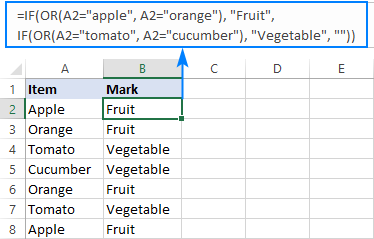### Formula 5. IF AND OR statement

To evaluate various combinations of different conditions, you can do AND as well as OR logical tests within a single formula.

As an example, we are going to flag rows where the item in column A is either Apple or Orange and the quantity in column B is greater than 10:

`=IF(AND(OR(A2="apple",A2="orange"), B2>10), "x", "")`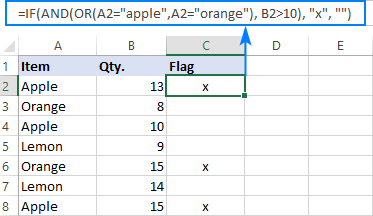That's how you use IF and OR functions together. To have a closer look at the formulas discussed in this short tutorial, you are welcome to download our sample Excel IF OR workbook. I thank you for reading and hope to see you on our blog next week!

## You may also be interested in

1. In Colum H I have a weekly cost 398.70
in Column I - I want to be able to put in a "Y" or an "N".
I want the Y to be half the amount of 397.70 (meaning apportioned 1:2 so it would be 199.35)
I want the N to be 398.70 (meaning the full amount not apportioned)

2. Hi there, im trying to give a single cell a number value based off 2 words. Ive tried the IF but cant seem seem to figure it out. I want
Cell F3 to give a number 2 if its a "W" and cell F3 also to give me a -1 if the text is a "L". Ive done IF(f3="w",2) and it works but cant figure out to add the "L" portion to the formula

3. Hey, so I am having troubling writing an if statement. I tried using concrate with it also so I can reflect two different outcomes. But everytime I create a formula it only reflects one option of what I wanted.

For example I was using :
=if(or(e1=“sw turnstile in”,”se lby turnstile in”, “in”,”out”),if(or(e1=“sw turnstile out”,”se turnstile out”,”out”,”in”)))

• Hey, read carefully in the article above how to use the OR function correctly. For example,

OR(e1=“sw turnstile in”,e1=”se lby turnstile in”, e1=“in”,e1=”out”)

4. I need a "IF" formula for

If 10mm then (-)1
If 15mm then (-)1.25
If 20mm then (-)1.75

5. Hello,

I am stuck and could really use some help.

On my worksheet that I track e-commerce sales I have each unique item in my store listed, 1 per row in a table. When an item sells the date sold is recorded. All sales are then taken to the post office that day. Some days I might have 2 sales and the next may have 30 sales. I want to try and calculate my cost of fuel divided by the number of shipments I have that day to disperse the overhead evenly. Since the post office is closed on Sunday all sales for Sunday are combined into Monday.

How can I state that IF date sold = Sunday Then Fuel Cost is zero, (AND/OR) Monday = Sunday sales + Monday Sales, (OR) Tuesday - Saturday are straight countIF calculations?

From some of your other tutorials I came up with the following: =IF(WEEKDAY([@[Date Sold]], 3)<6, (3.5/(COUNTIF(L:L,[@[Date Sold]]))),"0")

For this example: \$3.50 is the fuel cost each trip.
Column L is DATE SOLD so once it sells this is populated with that date.
Column W is where I am trying to capture fuel cost, per item where this formula currently lives. It works for returning a proper result (except for Monday) I just don't know whether this is an OR, AND, nesting solution and where to place the arguments? I also cannot seem to get SUMIF to add by groups of dates. Is that even possible to do?

I have tried several combinations of IF & IFS, but I can't seem to get the right order to get Monday to take Sunday and Monday together. I have also toyed around with WORKDAY & WORKDAY.INTL per those tutorials but I obviously don't understand them well enough because I just kept getting errors.

• Hello! To combine Sunday and Monday, use an expression like this: WEEKDAY(D1,12)>5

6. Hi all,

Good day! Hoping everyone had a great day! Please i need help on a formula. I want to display the result "ASP" if the starting letter starts with ID, IN, CN etc (whatsoever that I declare as long as it starts with 2 letters) otherwise "NON-ASP" for those 2 starting letters not declared on the formula.

Samples
ID0523A2292
IN623PEA-3666
TW623PEA-3677
CN0623MXA-1447
CN0623MXA-1343
IN0623MXA-1438
ID0623MXA-1471
VN0623A2382
VN623PEA-3696
VN623PEA-3697
VN623PEA-3698
CN0623MXA-1518AND

Thank you very mych

• Hi! If I understand correctly, extract the first two characters from the text string and perform a math operation on them. The ISERROR function will indicate an error if the value is a letter. Try this formula:

=IF(AND(ISERROR(--LEFT(A1,1)), ISERROR(--MID(A1,2,1))), "ASP", "NON_ASP")

• Hi Alexander,

The samples I gave were the actual identifications from my work. If the identification starts with ID or CN or VN or TH (many more combinations) then it will display "ASP" otherwise if not declared then it displays "NON-ASP".

ID0523A2292 - this one should display "ASP" since the 2 starting letter is ID
CN0623MXA-1447 - this one should display "ASP" since the 2 starting letter is CN
MX6756VN-123 - this one should display "NON-ASP" since the 2 starting letter is MX

Thank you,

• You would have saved your time and my time if you had described the problem in detail and accurately at once. Use this formula, where H1:H10 is the list of allowed values.

=IF(ISNUMBER(MATCH(LEFT(A1,2),H1:H10,0)),"ASP","NON_ASP")

7. Dear Alexander,
I hope you are doing well,

I have one question and i need an excel formula for that if possible ,please,
I have to generate a quarterly report for a real estate company and i have contracts for flats, shops, warehouses etc....
So what exactly i want is to make sure if the tenant contract is valid during the report period and how many days is the validation?
For Example,
The reporting period is from 01.01.2023 Until 31.03.2023.
The flat contract period is from 22.02.2022 until 21.02.2023.
So the period i want the excel formula to calculate it is the report period validation during the contract of the flat contract period i mean the period will be from 01.01.2023 Until 21.02.2023 which is "one month which is January+ 21 day from February.
Could you tell me how can i do excel function that generates the return of "1month +21 days"

8. Want to identify cases in 4 categories, values are in number format, however the IF logic is not giving correct answer,
Expected answer is derived from filter logics at frontend, have created 4 slabs 1 day
have used 3 different formulas however not able to get desired output, Please help to make corrections

test = IF(B3<1,"=1,B3=4,B3<24),"4 to 24 Hrs","test")))
test1 = IF(B3<1,"=1)+(B3=4)+(B3<24),"4 to 24 Hrs","test")))
test2 =IF(B3<1,"=1 + B3=4 + B3=24,"> 1 Day"))))

0.05 < 1 Hour 1 to 4 Hrs <1 Hour <1 Hour
0.09 < 1 Hour <1 Hour <1 Hour 1 Day <1 Hour <1 Hour 1 Day 1 to 4 Hrs <1 Hour <1 Hour
1.02 1 - 4 Hours 1 to 4 Hrs <1 Hour <1 Hour
1.16 1 - 4 Hours 1 to 4 Hrs <1 Hour <1 Hour
22.68 4 Hr to 1 day 1 to 4 Hrs <1 Hour <1 Hour
4.48 4 Hr to 1 day 1 to 4 Hrs <1 Hour <1 Hour

• used below formula also with OR condition,

=IF(BE2<1,"=1,BE2=4,BE2<24),"4 to 24 Hrs","test")))
however not matching with expected output

9. I need help with this formular on excel. Not sure if to use the actual colmmn names. eg Column DK is EBL, DL is CARB and DM is AMP.

Trying to create a new column called GenTY

where if DK is yes and DL is yes and DM is yes then GenTY = "CarbR".
If only DK is yes then GenTY = ESBL-G
If all are "no" then GenTY = "Negative" and so on.

=IF(OR(DK="yes", DL="yes", DM="yes"), "CarbR", IF(OR(DK="yes", DL="no", DM="no"), "ESBL-G", IF(OR(DM="yes", DL="no", DK="no"), "AmpCG", IF(OR(DK="yes", DL="no", DM="yes"), "ESBL/Amp", IF(OR(DM="no", DK="no", DL="no"), "NEG"))))))

• Hi! I don't understand what you want to do. If we're talking about named ranges, they can't be created with a formula. Your formula doesn't look like a normal Excel reference.

• Hi Alex, I wanted to use Excel to create a new column in my data set based on a set of conditions. The new column will be created based on variables in columns DK, DL and DM.

The new column is called DN.

So when the value in "DL" is yes then "DN" = "CarbR".
If only DK is yes then "DN" = ESBL-G
If only "DM" is yes then "DN" = AmpC
If either of the column is "no" then "DN"= "Negative".

I hope you understand my question now.

Thanks

• I don't understand what "column is called DN" means. Read carefully the article on references in Excel and named ranges.

10. Hi,

Having a bit of a problem

Sheet 1
1 = 5
2 = 10
3 = 15

Sheet 2
Enter either 1, 2, 3 and get the corresponding values

This works when I enter 2. =IF(C11=2,(SoR!F13))
This doesn't work when I enter 3 but works when I enter 2. =IF(C12=2,(SoR!F13))*OR(C12=3,(SoR!F19))

• Thank you for your help Alexander.

This is my problem. Sheet 1 may have up to 100 entries and I want to select these when I enter the number in a cell in sheet 2

Sheet 1

Item Reference Value
1 10.00
2 20.00
3 30.00
4 40.00
5 50.00
6 60.00
7 70.00
8 80.00
9 90.00
10 100.00
and more

Sheet 2 Input Sheet

Enter any reference from sheet 1 Corresponding Value from sheet 1
1,2,3,4,5,6,7,8,9,10 10,20,30,40,50,60 etc

How do I create a formula for 10 or more items

• I need to send you copies of the spreadsheets. How do I do that?

11. Hello all,

I appreciate any help.

I'm looking to create a document that can produce multiple values depending on the parties involved and documentation requested from each. For example.
If B2 = A then I need X documents
If B2 = B then I need Y documents
If B2 = C then I need Z documents
If B2 = ... the I need ... Documents so on and so forth for about 10 different scenarios. 10 different possible parties with 10 different possible document names.

Is this possible? I just keep getting "you've entered too many arguments for this function"

Gratefully - Hoyt

12. Please help me out (if one column is Paid or unpaid with conditional formatting then one cell value decreases with the other column amounts how can i use if formula in this scenerio?)

• Please clarify your specific problem or provide additional details to highlight exactly what you need. As it's currently written, it's hard to tell exactly what you're asking.

13. HI there,

Been having a hard time getting the hang of this but what I'm trying to do is this:

IF(E10="", "", IF(OR(E10="TOM", E10="BEN", E10="JOY"), 5%, 8%))
----

I wanted to leave the cell "blank" if there's no detail encoded in E10 if not it should check if any of the names matches the list it would result to 5% interest rate, otherwise 8% only.

• Hi! If I understand your question accurately, this formula works correctly. If there is a problem, describe it in more detail.

• It works but somehow it doesn't apply the 5% interest rate if the cell contains any of the mentioned names. It always return the value of 8%. Not sure why it doesn't end up to 5% if all conditions are met.

=IF(ISBLANK(E10), "", IF(OR(E10="BEN", E10="JOY", E10="TOM"), 5%, 8%))
-----

Tried the above formula but it always result to 8%. I intend to leave the cells blank as much as possible in order not to appear in the printable. If I switch the position of interest rate, the value in "if_value_false" always the end result.

• Thanks sir Alex,

I've finally figured out my mistake, it appears that I made the wrong cell reference for my OR Argument, it should be linked to the cell that contains a list of names, that is why it doesn't work function I wanted it to be.

=IF(OR(D5="TON", D5="BON", D5="JOY"), 5%, IF(ISBLANK(E5), "", 8%))
-------

Using the formula above solved my problem...

Thank you so much for this site :)

• Hi! The formula works correctly. Check cell E10. Maybe there are extra spaces or other characters.

14. What if you want a function to calculate if you gonna times by 30 if someone is on self catering(C2) of by 95 if the are B&B catering(C3) if you are given number people in cell C7 are 6 people and number of nights they are 7 on D7 and someone is on self catering but you want a formular so that you can calculate those that are on B&B

15. I can get this =IF(OR(F2="Awaiting Shop",F2="In Shop"),"VMS","") formula to work but I need to also look at a Table to see if it is somewhere else.

I tried the following that produced a #VALUE! Error
=IF(OR(F7="Awaiting Shop",F7="In Shop"),"VMS",IF(VLOOKUP(@A:A,Location,3,FALSE),""))

Column A has the Name of the truck I'm looking for in the Table named Location, the information I looking for is in the 3rd column of that Table.

Is this possible to do at all?

I Tried =IF(OR(F2="Awaiting Shop",F2="In Shop"),"VMS","") in the table but the table has drop downs and wont work.
Also Tried this formula in the dropdown but produces a 0 in the sheet that Need the information.

Any ideas?

16. i have 3 worksheets of part numbers. i want to look up a part number from sheet 1 and see if they exist on sheet 2 and sheet 3, if it does i want it to return a yes. i am a newbie with excel and cannot figure out the formula for this.

17. Hi Alexander,

i have the following IF/OR formula i'm try to build. Basically its looking up a cell for either ME (Meter),IN (Inch) or CM (Centimeter). if ANY of the dimensions are greater than 1.2 (ME), 120(CM) or 47.2 (IN) there is a value of 70. if not, the value is zero.
can you see where i'm going wrong on this

=IF(OR(AP3202="ME",AP3202>1.2,AQ3202>1.2,AR3202>1.2),"70","0"),IF(OR(X3202="CM",AP3202>120,AQ3202>120,AR3202>120),"70","0"),IF(OR(X3202="IN",AP3202>47.2,AQ3202>47.2,AR3202>47.2),"70","0")

help much appreciated.

thanks,
Damien

• Hi!
I do not have your data, so it is difficult to understand your formula and impossible to check it. However, check in the first OR condition: AP3202="ME",AP3202>1.2

18. Hi I am trying to get a formula for this:

-produce the label 'points if either a reservation's Total Tour Price exceeds 300 or has more than six(6) persons in the tour party. Otherwise leave the cell blank

I tried but getting it incorrect. Thanks

• Hi!

19. Hi there

Please Suggest I have a few criteria

There are 3 column A= Inventory on Website, B= Inventory In Hand and C= reserved qty (B-5)

Need to write a formula where, If A is greater than C then need to correct Website inventory and if C is 0 or less than 5 then update as out of stock and if C is greater than A and greater than 5 then, update inventory

20. This is great article & very helpful,

I am a beginner and tried to correct one of below formula on my own and it takes time.

=IF(C5="Mon";" ";OR(B11="Apple";B11="Banana");C11*1))

Or other way

=IF(C5"Mon";OR(B11="Apple";B11="Banana");C11*1;" ")

in C5 I have name of the day like Mon, Tue, Wed etc.
in B11 I have a fruit names like Apple, Banana, Grapes etc.
in C11 I have number of kilo

I tried several combinations of the parentesis as well as AND, NOT functions but no luck.

this is the problem;
- if it is a "Mon" don't calculate kilos of these fruits.
- if it is not a "Mon" calculate kilos of only these fruits

if is this kind of formula possible for kind of problem? and would you please help on this.

Thank you very much in advance

• Hi! Based on your description, it is hard to completely understand your task. However, I'll try to guess and offer you the following formula:

=IF(AND(C5="Mon"; OR(B11="Apple";B11="Banana"));"";C11)

If you want to calculate the sum for these fruits, use the SUMIFS function. I recommend reading this guide: Excel SUMIFS and SUMIF with multiple criteria – formula examples.

• Hi! Alexander,

The above formula did not worked thru but I add one more if(..) in the middle and it worked

=IF(AND(C5="Mon",if(OR(B11="Apple";B11="Banana"));"";C11)

Thank you very much.

21. Hi,

I have 6 variables in total. Only one variable will actually be found each time and then I would like that specific variable back in text.
*have to use ";" instead of comma's in my excel.
Doing this now, but not working:

=IF(OR(ISNUMBER(SEARCH("Var1";A28));"Var1");
IF(OR(ISNUMBER(SEARCH("Var2";A28));"Var2");
IF... etc. ))

• Hello!
You can use nested IF formula

=IF(ISNUMBER(SEARCH("Var1",A28)),"Var1",IF(ISNUMBER(SEARCH("Var2",A28)),"Var2",IF(ISNUMBER(SEARCH("Var3",A28)),"Var3","")))

If you have many conditions try using the IFS function instead of multiple IF:

=IFS(ISNUMBER(SEARCH("Var1",A28)),"Var1",ISNUMBER(SEARCH("Var2",A28)),"Var2",ISNUMBER(SEARCH("Var3",A28)),"Var3")

22. Hi need help. I would want to automatically get the rates when these combinations are selected. Please see table. Thank you so much in advance.

Service Paper Size Print Color Rate
Print - Plain TEXT Letter Grayscale | B/W 5.00
Print - Plain TEXT A4 Grayscale | B/W 5.00
Print - Plain TEXT Long / Folio Grayscale | B/W 7.00
Print - IMAGE (Half page) Letter Grayscale | B/W 7.00
Print - IMAGE (Half page) A4 Grayscale | B/W 7.00
Print - IMAGE (Half page) Long / Folio Grayscale | B/W 10.00
Print - IMAGE (Half page) Letter Colored 12.00
Print - IMAGE (Half page) A4 Colored 12.00
Print - IMAGE (Half page) Long / Folio Colored 15.00
Print - IMAGE (Full page) Letter Grayscale | B/W 10.00
Print - IMAGE (Full page) A4 Grayscale | B/W 10.00
Print - IMAGE (Full page) Long / Folio Grayscale | B/W 12.00
Print - IMAGE (Full page) Letter Colored 15.00
Print - IMAGE (Full page) A4 Colored 15.00
Print - IMAGE (Full page) Long / Folio Colored 20.00
Print - Digital photo 4R Colored 30.00
Photocopy Letter Grayscale | B/W 5.00
Photocopy Letter Colored 7.00
Photocopy A4 Grayscale | B/W 5.00
Photocopy A4 Colored 7.00
Scan 10.00

• Thank you for this but I am not looking for the delimiter. What I am trying to get is the "RATES". For instance if I input "Print - Plain TEXT" on "SERVICE" then "Long / Folio" on the "PAPER SIZE" then "Grayscale" on "Print colour" it will get me automatically the "RATE" of 7.00..

• Hi!
From text: Print - Plain TEXT Long / Folio Grayscale | B/W 7.00 - formula extracts 7.00
If it is not a single text string but several cells, which you did not mention, use these guidelines: Excel INDEX MATCH with multiple criteria.

• Hey Alexander Trifuntov ! Thank you so much for the help. Works really great! Awesome! Just as the result I really wanted. :D

23. I am trying to sum a range of cells if another range of cells says either yes or no. If yes then sum the cells, if no, then subtract the amount in that cell. Can someone help?

24. doc_no frm_date to_date missing date
1662450337 01-Apr-22 04-Apr-22
1662450337 05-Apr-22 07-May-22
1662450337 08-May-22 04-Jun-22
1662450337 05-Jun-22 04-Jul-22
1662450337 05-Jul-22 04-Aug-22
1662450337 05-Aug-22 04-Sep-22
1662450337 05-Sep-22 04-Oct-22

25. Hi,

=IF(OR(K20="DE",K20="FR",K20="SE",K20="ES",K20="IE",K20="IT",K20="DK",K20="NL",Z20>150),"GB 320000",K20)

if for example K20= "CH" is not listed in the above formula. is there an add on to this formula to just show K20 as CH

Hope this makes sense.

thanks

• this is the full formula i'm looking for, but no joy. help would be greatly appreciated

=IF(\$K20="DE",IF(\$Z20>150,GB 320000),IF(\$K20="FR",IF(\$Z20>150,GB 320000),IF(\$K20="SE",IF(\$Z20>150,GB 320000),IF(\$K20="ES",IF(\$Z20>150,GB 320000),IF(\$K20="IE",IF(\$Z20>150,GB 320000),IF(\$K20="IT",IF(\$Z20>150,GB 320000),IF(\$K20="DK",IF(\$Z20>150,GB 320000),IF(\$K20="NL",IF(\$Z20>150,GB 320000),IF(\$K20="CH",IF(\$Z20>0,CH)

• Hi!
Add another OR condition as described in the article above.

26. I am using the following formula, but I am finding examples where the SUM of T to V = 2 in the first argument and it is still returning a Compliant result when it should be Non Compliant for not being = to 3?

=IF(OR(N46=1,SUM(\$T46:\$V46)=3,N46=0,SUM(\$T46:\$U46)=2),"Compliant","Non Compliant")

• Hello!
You are using the logical OR function. If at least one condition is true, the formula returns TRUE.

• Simple formula, but I can't figure out how to use IF, or if it is IF OR or IF AND to nest the ifs.

Column A (Salary) has values ranging from 10 to 100.
I want to indicate in Column B whether the numbers in Column A would be, '75 and below,' '50 and below,' and '25 and below.'
I can do the basic =IF(A2<=100,"100 or less","0") but then get stuck trying to add the '75 and below,' '50 and below,' and '25 and below.'
Thanks!

27. Hi,

Thanks for you great works.

I am working on a file with column A containing dropdown list of numbers 100, 200, and 300. The number represents "account department", "legal department" and "sales department" respectively.
How can I make column B dependent on what is chosen on the dropdown list of column A? That is, if 100 is chosen on the dropdown list in column A, I want column B to return "account department" on its own.

Thank yoy.

28. I would like to calculate a sum of products, but with a pricing break.

1st item= \$50, 2nd item onwards = \$70 each

Let's say if A buys 3 products, he will have to pay \$50 for the first product, for the other 2 items, he will have to pay \$70 each.

How could I create a formula for this problem? Hope you could assist me, it'll be a great help

• Hi!
Use the IF function to calculate the sum for values greater than zero.
The formula below will do the trick for you:

=IF(A1>0,(A1-1)*70+50,0)

29. I'm trying to use IF to show "ok" or "out of balance" if a value is over or under by more than 5%.
Cell C20 has a value of 700
Cell C21 has a value of 650
My formula for D20 is =C20-C21 giving a value of 50
My formula for D20 is =IF(D20< C20*5%,"Ok","Out of balance")
This works but if the figures in C20 & C21 were reversed the value in D20 is -50 and shows as"Ok"
What I want is the formula to show "out of balance" if the value was over OR under by more than 5%

• Hi!
To ignore what is a positive or negative number, use the ABS function -

=ABS(C20-C21)